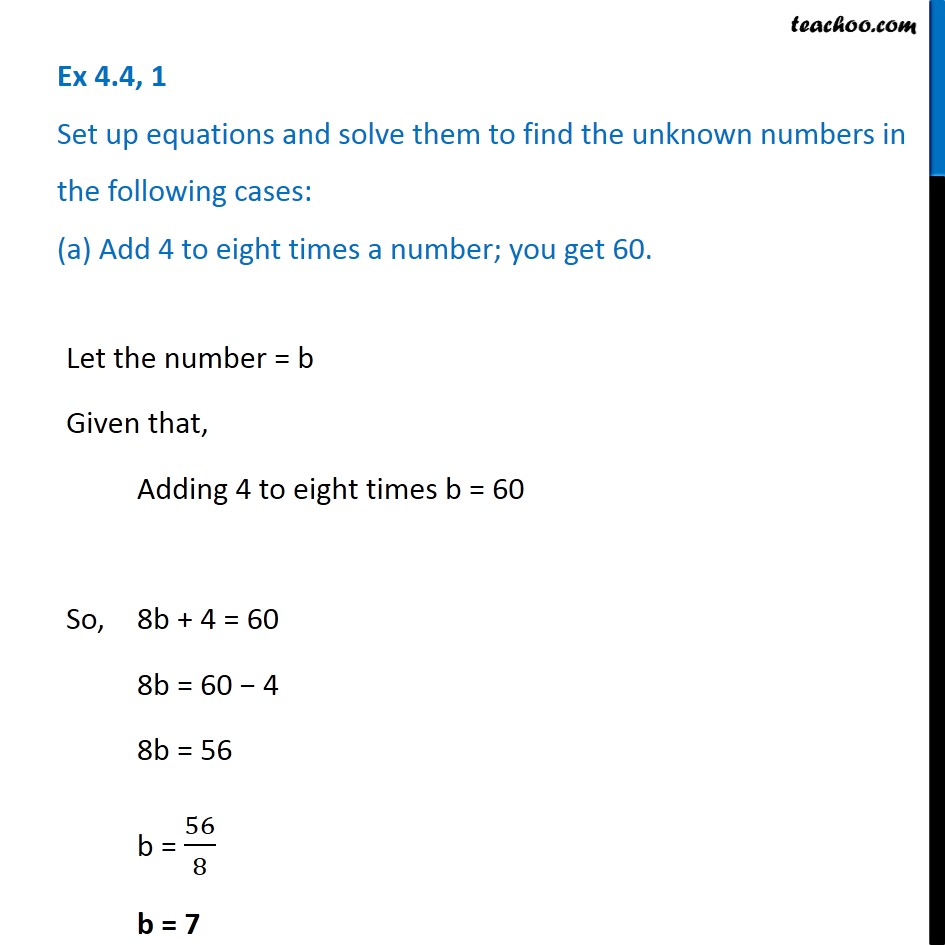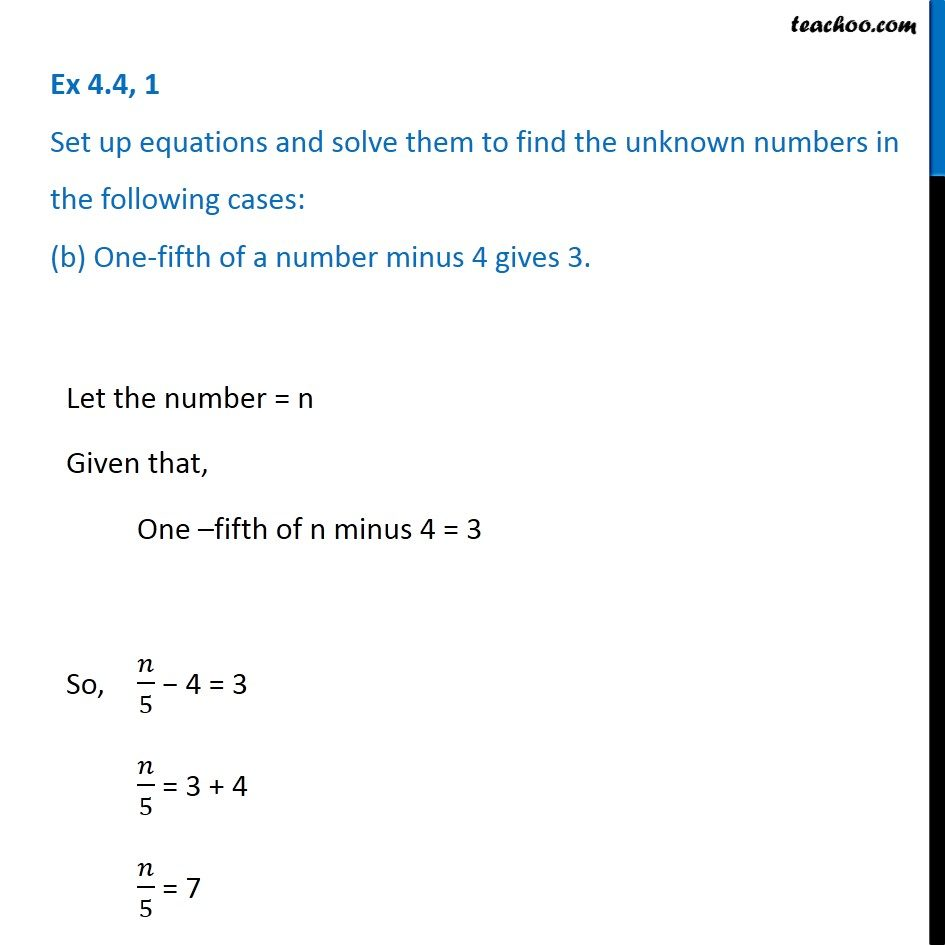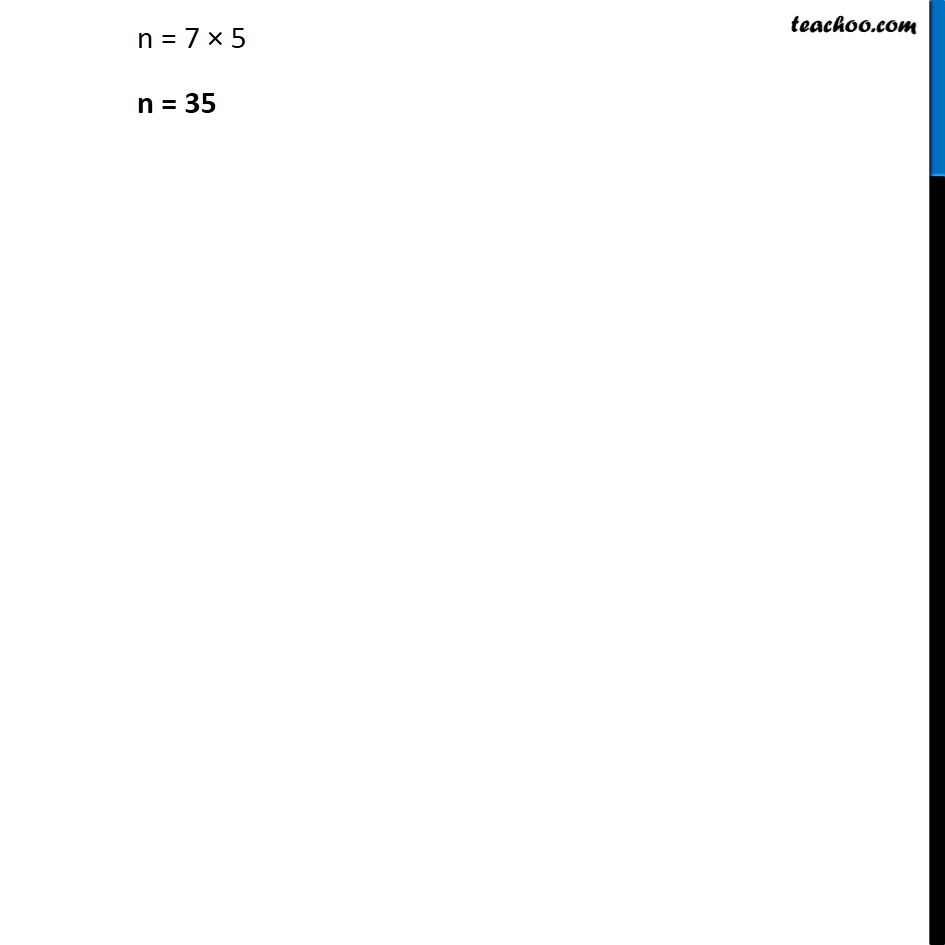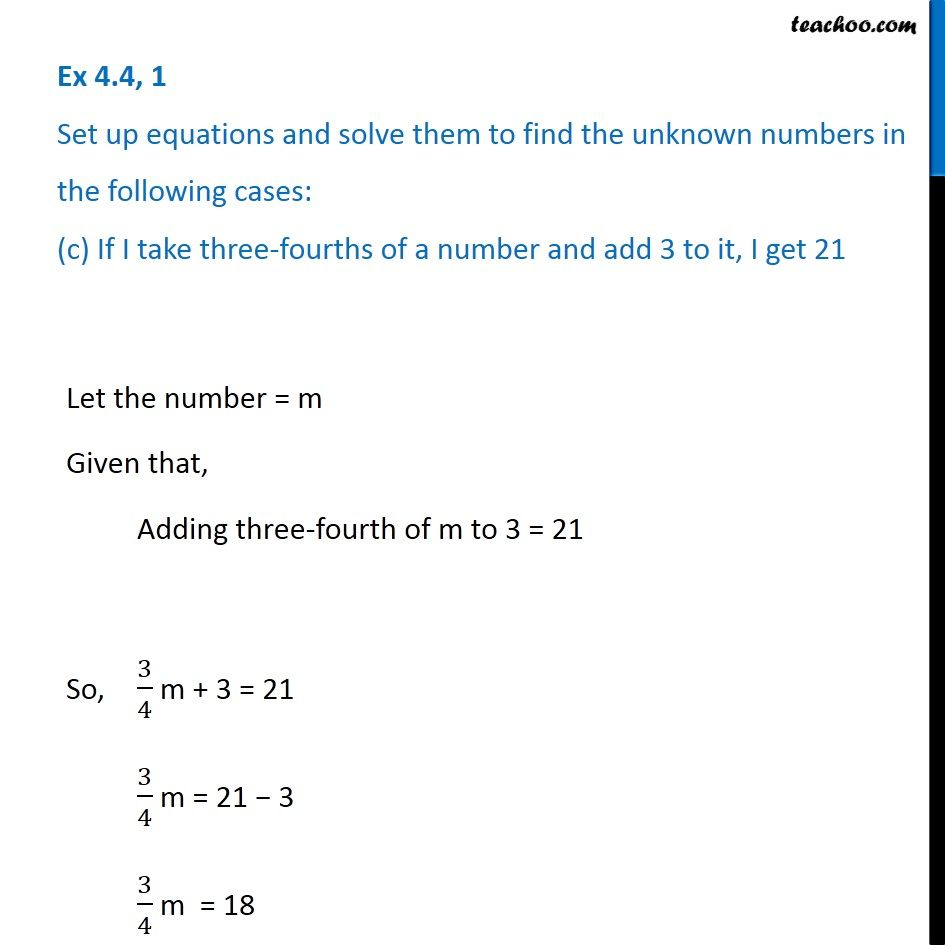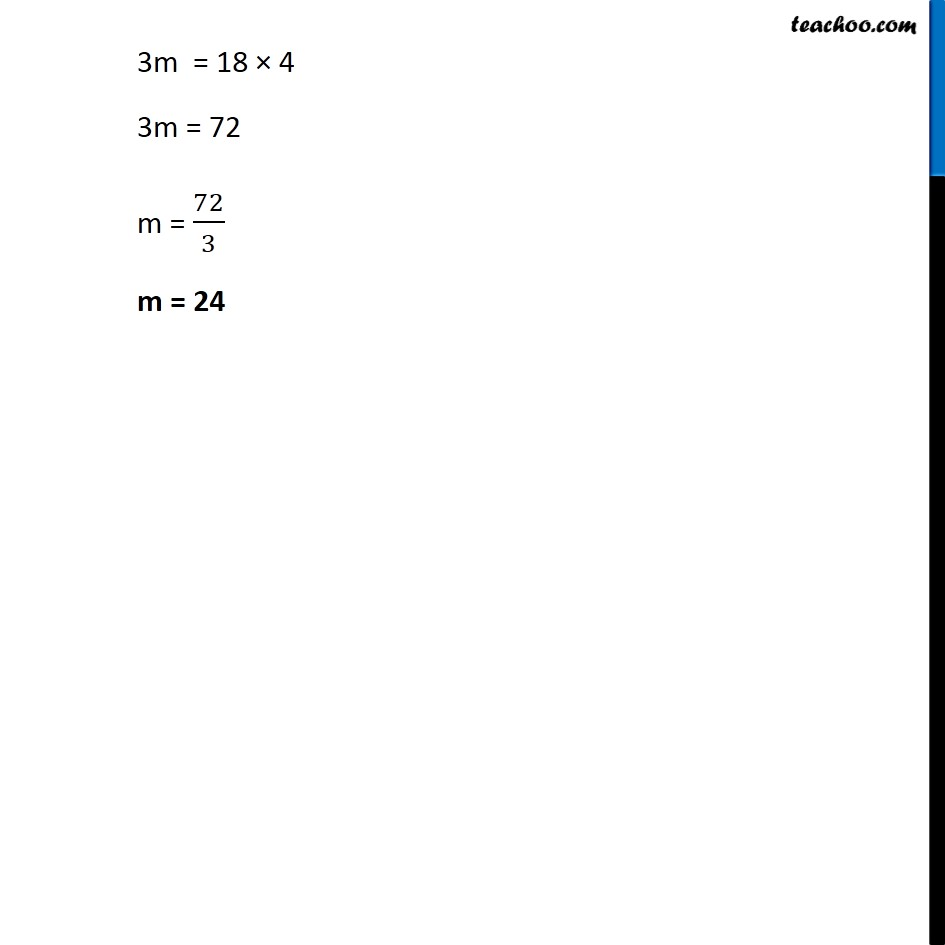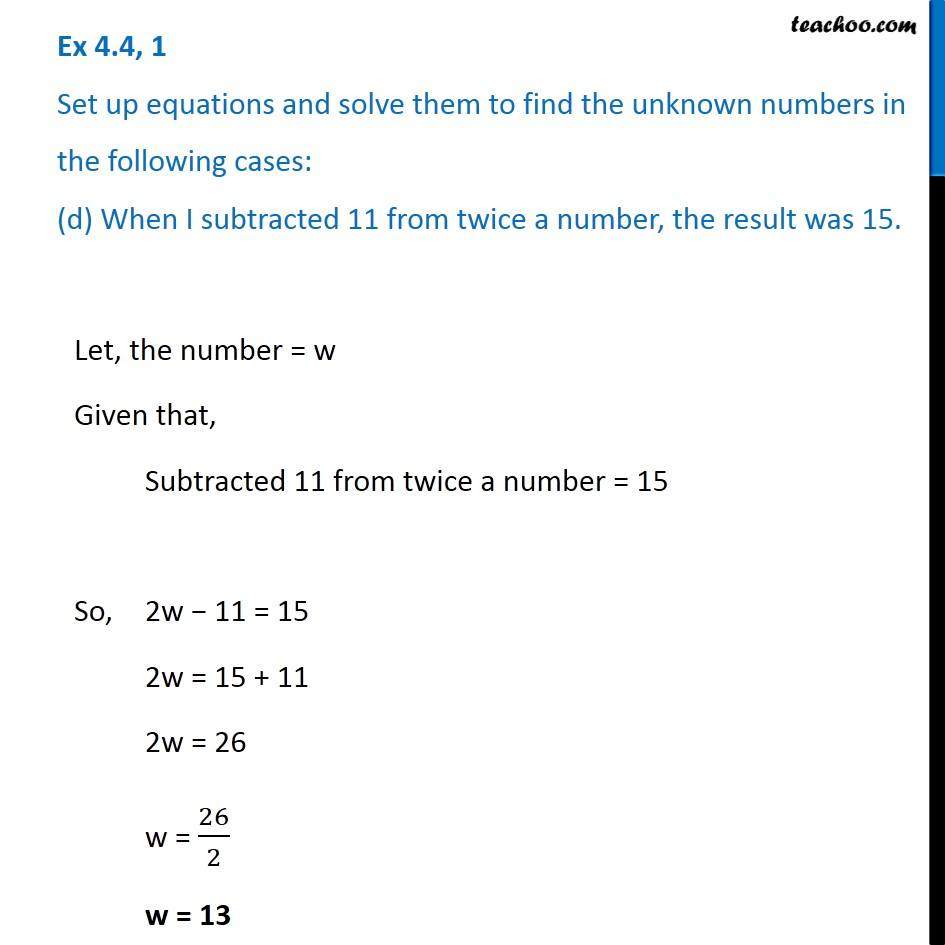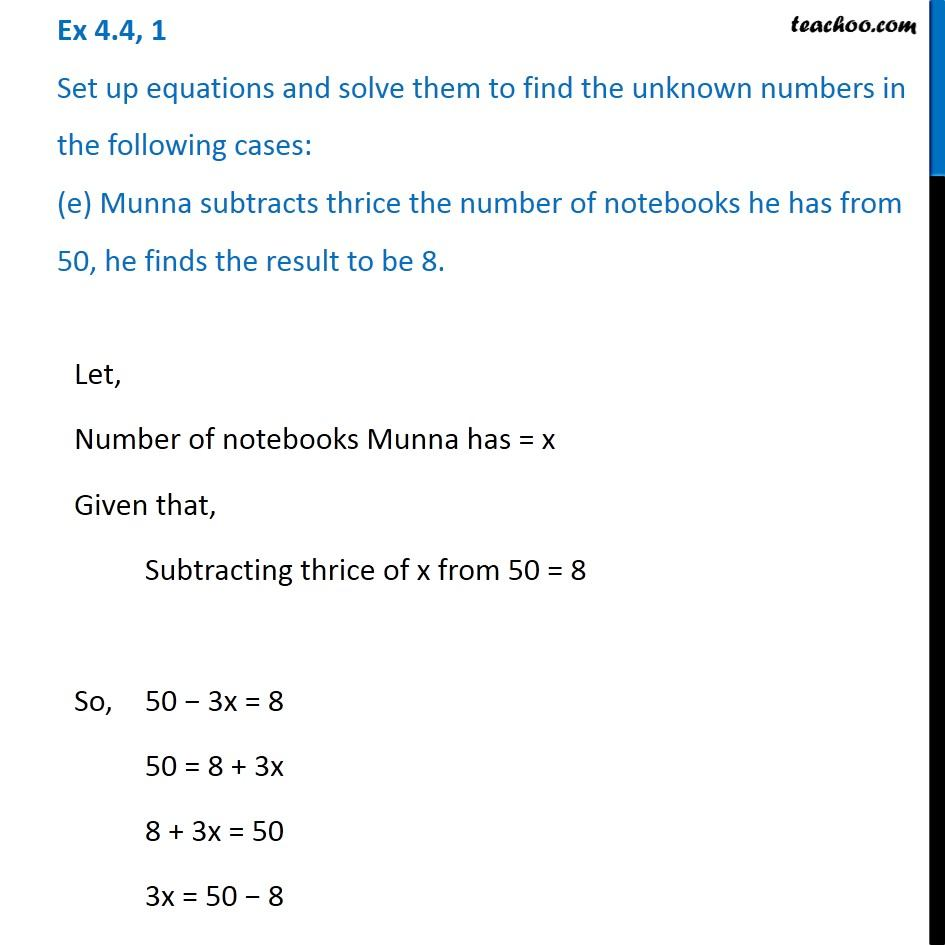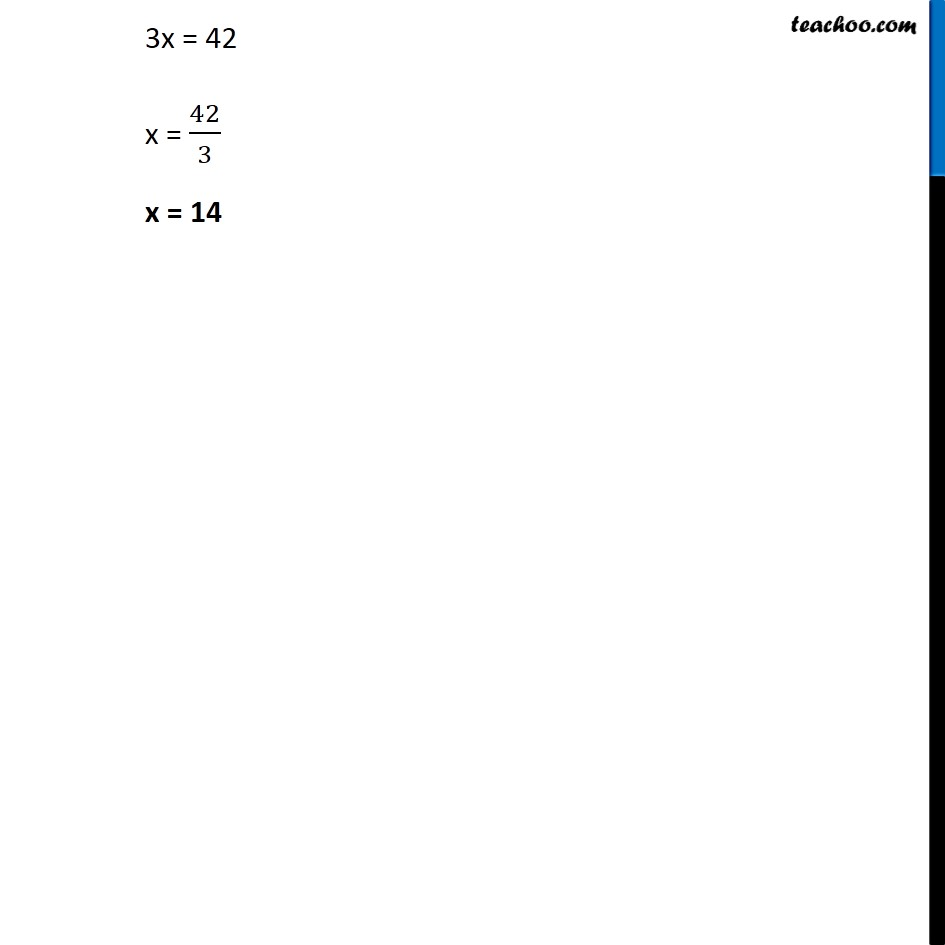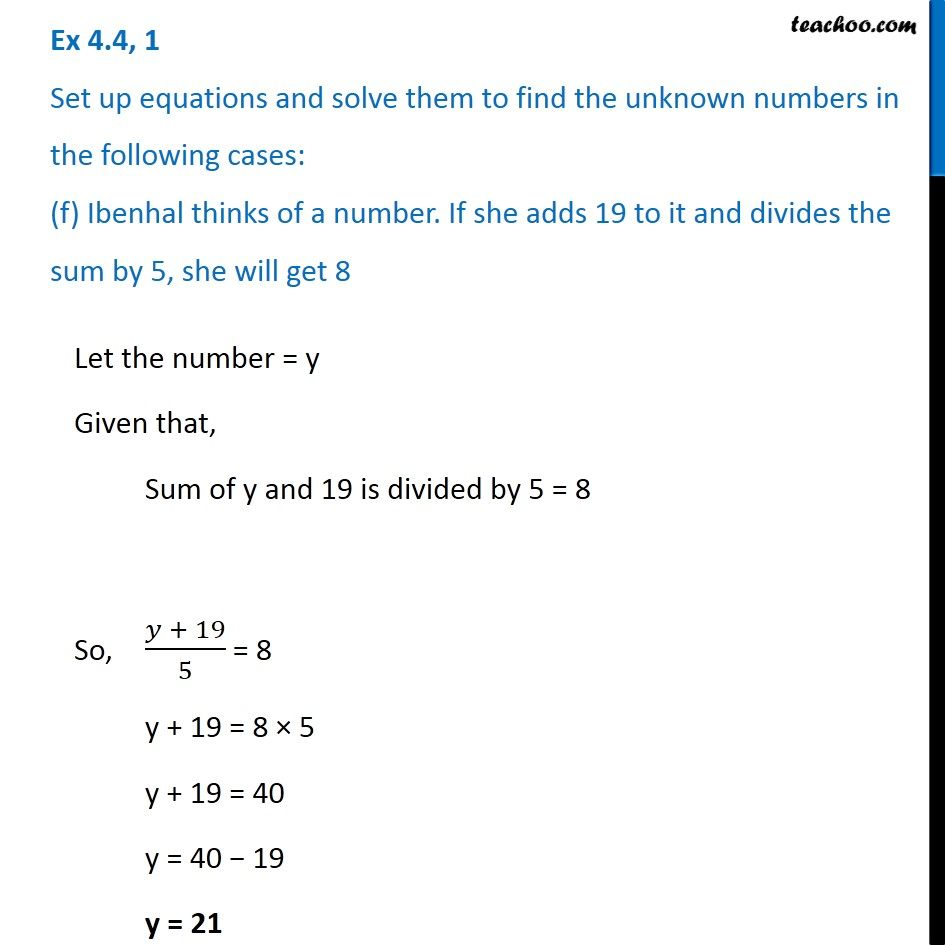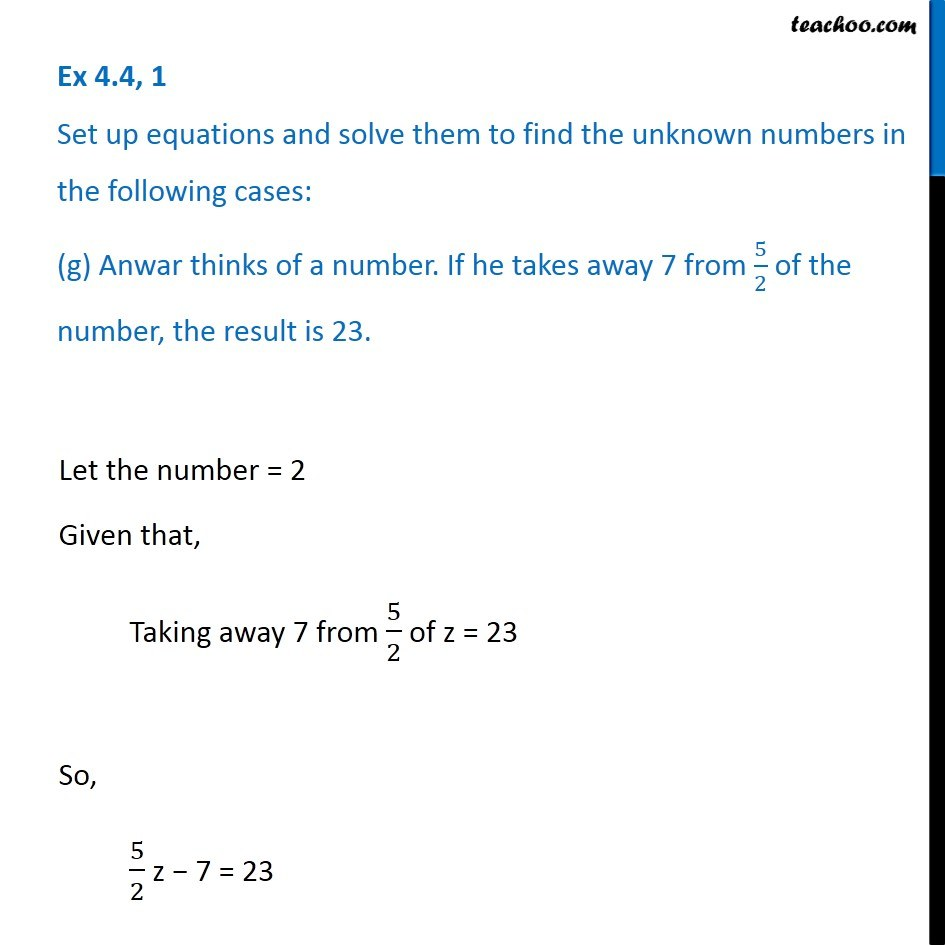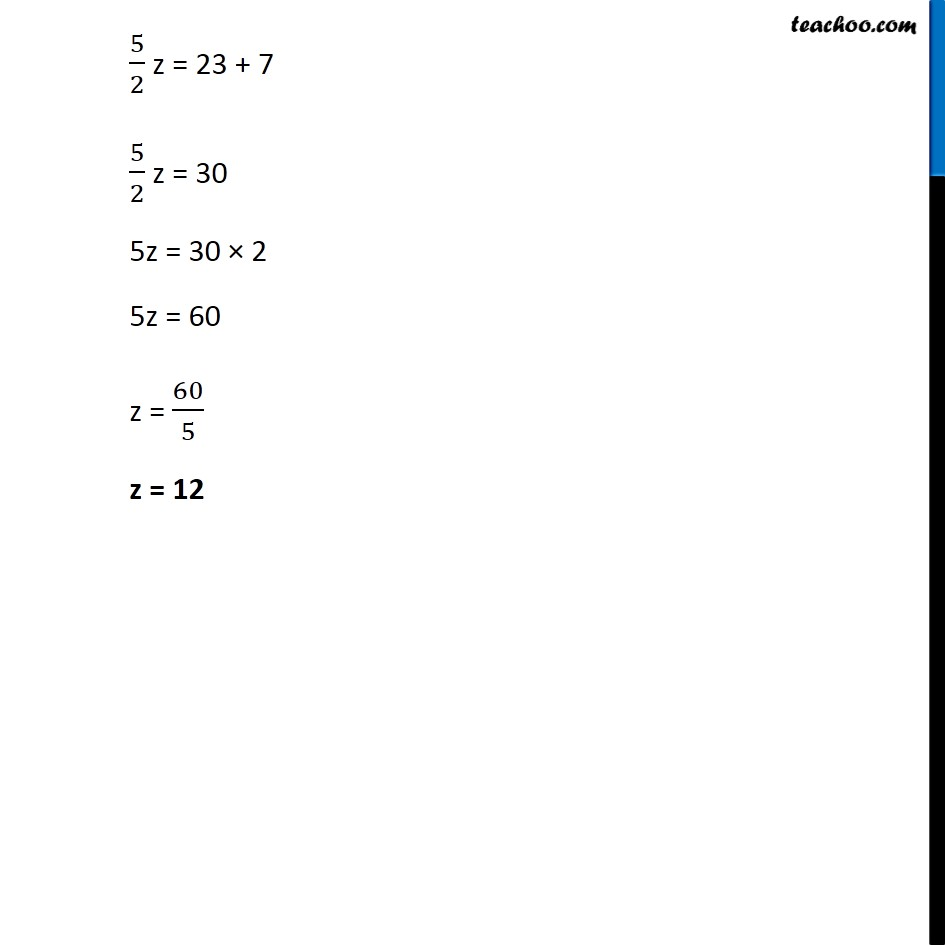Subscribe to our Youtube Channel - https://you.tube/teachoo

1. Chapter 4 Class 7 Simple Equations
2. Serial order wise
3. Ex 4.4

Transcript

Ex 4.4, 1 Set up equations and solve them to find the unknown numbers in the following cases: (a) Add 4 to eight times a number; you get 60. Let the number = b Given that, Adding 4 to eight times b = 60 So, 8b + 4 = 60 8b = 60 − 40 8b = 56 b = 56/8 b = 7 Ex 4.4, 1 Set up equations and solve them to find the unknown numbers in the following cases: (b) One-fifth of a number minus 4 gives 3. Let the number = n Given that, One –fifth of n minus 4 = 3 So, 𝑛/5 − 4 = 3 𝑛/5 = 3 + 4 𝑛/5 = 7 Let the number = n Given that, One –fifth of n minus 4 = 3 So, 𝑛/5 − 4 = 3 𝑛/5 = 3 + 4 𝑛/5 = 7 Let the number = n Given that, One –fifth of n minus 4 = 3 So, 𝑛/5 − 4 = 3 𝑛/5 = 3 + 4 𝑛/5 = 7 n = 7 × 5 n = 35 Ex 4.4, 1 Set up equations and solve them to find the unknown numbers in the following cases: (c) If I take three-fourths of a number and add 3 to it, I get 21 Let the number = m Given that, Adding three-fourth of m to 3 = 21 So, 3/4 m + 3 = 21 3/4 m = 21 − 3 3/4 m = 18 3m = 18 × 4 3m = 72 m = 72/3 m = 24 Ex 4.4, 1 Set up equations and solve them to find the unknown numbers in the following cases: (d) When I subtracted 11 from twice a number, the result was 15. Let, the number = w Given that, Subtracted 11 from twice a number = 15 So, 2w − 11 = 15 2w = 15 + 11 2w = 26 w = 26/2 w = 13 Ex 4.4, 1 Set up equations and solve them to find the unknown numbers in the following cases: (e) Munna subtracts thrice the number of notebooks he has from 50, he finds the result to be 8. Let, Number of notebooks Munna has = x Given that, Subtracting thrice of x from 50 = 8 So, 50 − 3x = 8 50 = 8 + 3x 8 + 3x = 50 3x = 50 − 8 3x = 42 x = 42/3 x = 14 Ex 4.4, 1 Set up equations and solve them to find the unknown numbers in the following cases: (f) Ibenhal thinks of a number. If she adds 19 to it and divides the sum by 5, she will get 8 Let the number = y Given that, Sum of y and 19 is divided by 5 = 8 So, (𝑦 + 19)/5 = 8 y + 19 = 8 × 5 y + 19 = 40 y = 40 − 19 y = 21 Ex 4.4, 1 Set up equations and solve them to find the unknown numbers in the following cases: (g) Anwar thinks of a number. If he takes away 7 from 5/2 of the number, the result is 23. Let the number = 2 Given that, Taking away 7 from 5/2 of z = 23 So, 5/2 z − 7 = 23 5/2 z = 23 + 7 5/2 z = 30 5z = 30 × 2 5z = 60 z = 60/5 z = 12

Ex 4.4